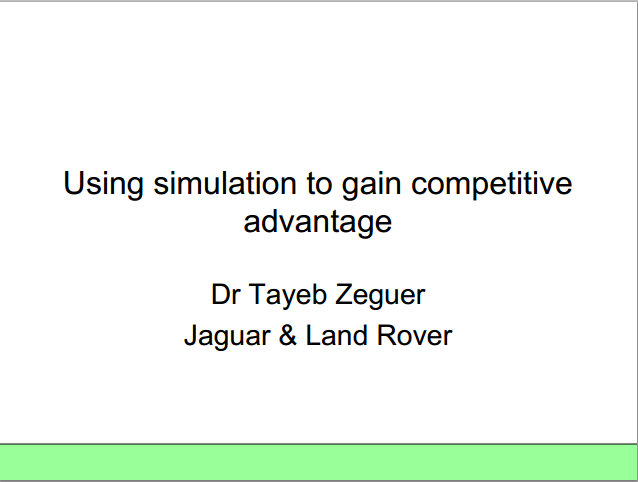# Using simulation to gain competitive advantage

Author:
Tayeb Zeguer (Jaguar & Land Rover)

After the Multi Objective Robust Design Optimisation (MORDO) an aggressive distribution was applied to the models by using UNIFORM distributions for each input variable as this maximizes the number of models with variables at the extremes of the tolerance ranges. Other distributions (normal, Cauchy, etc) would create a suite of models with a bias towards the nominal value. During the simulation many models (>200) were run with distribution around the nominal point. Of these runs, the number of models which pass all requirements (feasible) and the number of models which fail any (unfeasible) were used to create a ‘pass/fail ratio’. The ‘pass/fail’ ratio is a simple measure of robustness where high ratio is desirable as this indicates a robust setup.